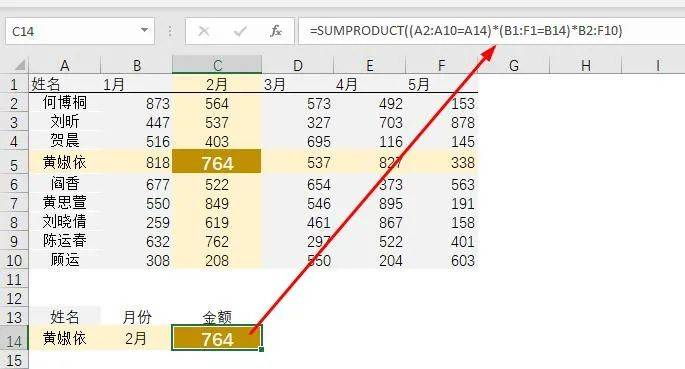# 这10个Excel“小”公式没人收藏，从此不再讲函数！

1、从月份中提取数字

=LEFTB(A2,2)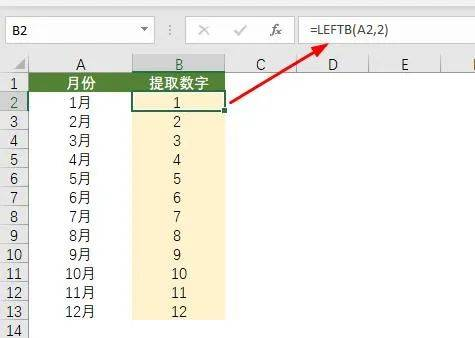2、跨多表同一位置求和

=SUM('*'!B3)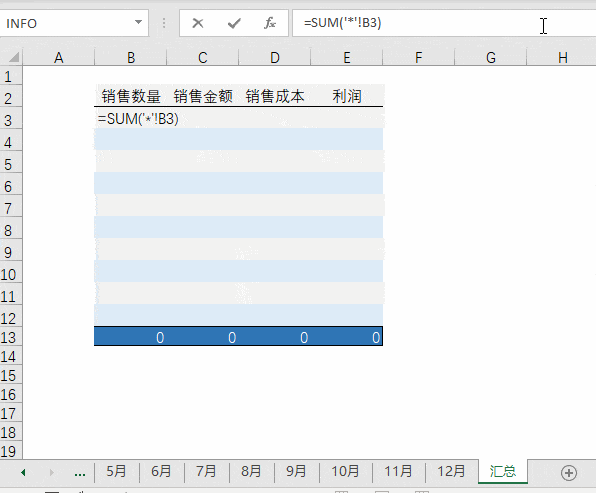3、总计公式

=SUM(B2:B20)/2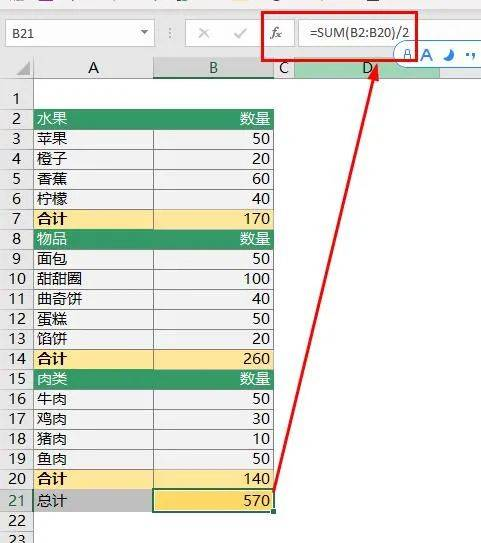4、合计单元格分类求和

=SUM(C2:C20)-SUM(D3:D20)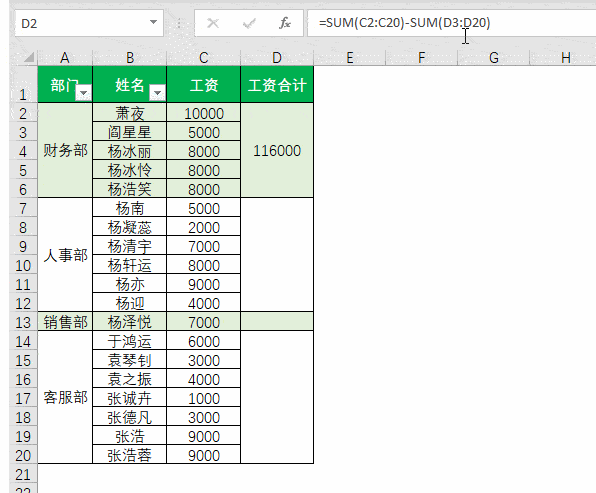5、限高取数

=Min(C4,2000)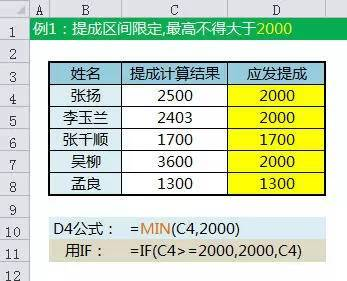=MAX(C4,200)

6、判断取值

101~105分别是"总办","销售","财务","客服","人事"对应的序号

C4公式：

=CHOOSE(B4-100,"总办","销售","财务","客服","人事")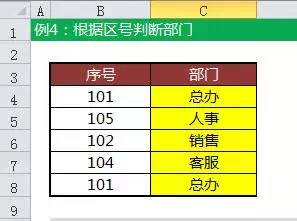7、身份证号计算个数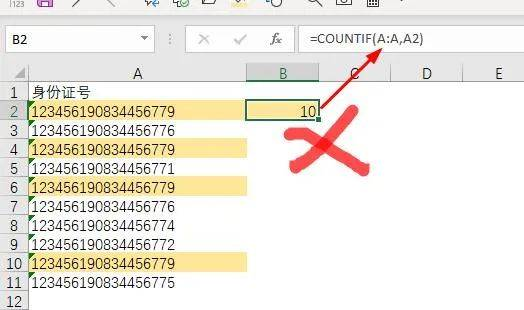=COUNTIF(A:A,A2&"*")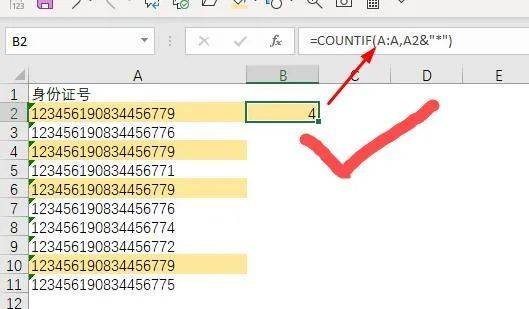8、从汉字+数字中提取数字

=MIDB(A2,SEARCHB("?",A2),99)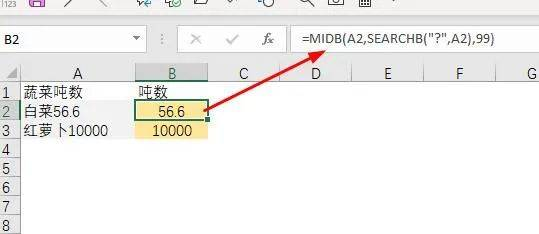9、从英文+数字中提取数字

=LOOKUP(9^9,--RIGHT(A2,ROW(1:99)))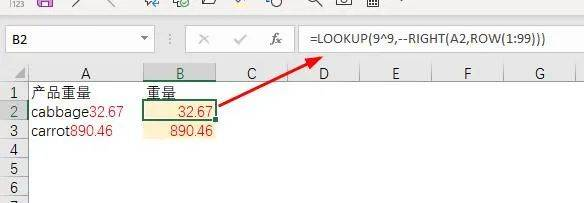10、双向查找

=SUMPRODUCT((A2:A10=A14)*(B1:F1=B14)*B2:F10)